# Expert GRE Probability Review: Formulas, Tips, and Practice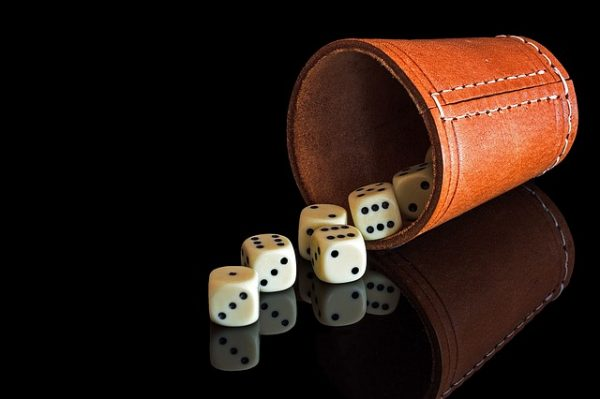What’s the probability of getting a perfect GRE Quant score? While you don’t have to be a math whiz to score highly on the GRE, you do need to be able to understand the basic concepts being tested, one of which is probability. Knowing what kind of GRE probability questions to expect will give you a little more confidence on Quant — and help raise your Quant score!

In this extensive GRE probability review, we’ll start by covering what probability is and the ways it’s presented on the GRE. Then, we’ll teach you all of the basic rules and formulas you need to know as well as give you tips for studying probability and approaching GRE probability questions on test day.

## An Introduction to GRE Probability

Probability is a sub-topic of data analysis, one of the four major math topics tested on Quant (in addition to arithmetic, algebra, and geometry). Unlike these larger topics, however, probability doesn’t play a significant role on the GRE. In fact, you’ll probably encounter only a couple of GRE probability questions in total — typically no more than two or three.

Still, it’s important you understand what probability is and how it’s often tested on the GRE. This way, you’ll be able to solve for the correct answer, ultimately raising your chance of achieving a high Quant score.

So what exactly is probability? In math, probability is a way for us to describe uncertainty and the possible outcomes of an experiment using numbers. These numbers indicate the likelihood of a certain event or group of events occurring and can be written as integers, decimals, or fractions.

On the GRE, probability questions can take on a variety of formats, including multiple choice, Quantitative Comparison, and Numeric Entry. Whether a question calls for fractions or decimals is usually clarified by the question or answer choices. Thus, a probability question discussing probabilities in decimals will probably ask for an answer in decimals. Answer choices, if supplied, are typically written either all in decimals or all in fractions.

If you are answering a Numeric Entry probability question, look closely at the blank to determine how you should write your answer. A single blank means the question is looking for either an integer or a decimal. A double blank (with a numerator and a denominator) means the question is looking for a fraction. (Note that you are not required to reduce fractions on the GRE; any equivalent fraction will count as a correct answer!)

Quick side note: we've created the world's leading online GRE prep program that adapts to you and your strengths and weaknesses. Not sure what to study? Confused by how to improve your score? We give you minute by minute guide.

You don't NEED a prep program to get a great GRE score. But we believe PrepScholar is the best GRE prep program available right now, especially if you find it hard to organize your study schedule and don't know what to study.

## GRE Probability: 16 Concepts You Must Know

You now have a fairly clear idea of how GRE probability questions look and are presented on the exam, so let’s move on to the math itself. What are the major terms, rules, and formulas you must know in order to understand GRE probability questions? Read on to find out.

### GRE Probability Terms

Below is a list of probability-related terms you’re most likely to encounter on the GRE:

• Random experiment: an experiment with an uncertain result
• Outcome: the result of a random experiment
• Event: a particular outcome or set of outcomes of a random experiment
• Sample space: the set of all possible outcomes of a random experiment
• Mutually exclusive: two or more events that cannot occur simultaneously
• Independent: when the occurrence of one event does not affect the probability of the occurrence of another event
• Random selection: the selection of an item from a sample space wherein all items are equally likely to be selected### GRE Probability Rules

Now, let’s learn about the math behind probability. Probability is usually denoted by P and can be written as P(E) to indicate the probability of a certain event occurring. The major rules are as follows:

• The probability of an event occurring always ranges from 0 to 1.
• If an event is certain to occur, the probability of that event occurring is equal to 1 and can be written as:

\$\$P(E)=1\$\$

• If an event is certain not to occur, the probability of that event occurring is equal to 0 and can be written as:

\$\$P(E)=0\$\$

• Most events fall somewhere within this range of 0 to 1 and have low, medium, or high chances of occurring. This concept can be written as an inequality:

\$\$0<P(E)<1\$\$

• To solve for the probability of an event not occurring, subtract the probability of the event occurring from 1. This looks like:

\$\$1-P(E)\$\$

• The sum of the probabilities of all possible outcomes of an experiment is always equal to 1.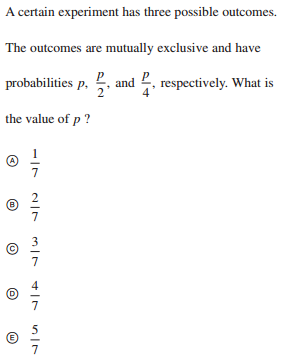Here, we have a multiple-choice probability question asking for an answer in the form of a fraction. In this question, a certain experiment contains three possible outcomes that are all mutually exclusive. (In other words, all of these outcomes must occur separately.) These three outcomes are p, \$p/2\$, and \$p/4\$.

According to the rules of probability, the probabilities of all outcomes of an experiment always add up to 1. In this case, the only three possible outcomes we have, denoted by their probabilities of occurring, are p, \$p/2\$, and \$p/4\$. Therefore, we can add all of these up to get 1, writing our equation as so:

\$p+{p/2}+{p/4}=1\$

Take a moment to examine the equation. Hopefully, you’ve noticed there is only a single variable in it: p. Because we’re being asked to find the value of p, all we have to do now is use algebra to simplify our equation and solve for p. The easiest way to do so is by multiplying each term by 4 and then combining like terms:

\$4p+{4p}/2+{4p}/4=4\$

\$4p+2p+p=4\$

\$7p=4\$

\$p=4/7\$

The correct answer is D: \$4/7\$.

### GRE Probability Formulas

Got your terminology down? Great! Now, let’s continue our GRE probability review by taking a look at the major probability formulas you’ll need for Quant.

#### For a Single Event

\$\$P(E) = {\number \of \successful \outcomes} / {\total \number \of \possible \outcomes}\$\$

• This is a basic formula and by far the most commonly used GRE probability formula.

#### For Two Independent Events

\$\$\Probability \of \two \independent \events \occurring = P(A) * P(B)\$\$

• P(A) = probability of event A occurring, P(B) = probability of event B occurring.
• Think of this equation as if you were rolling a regular six-sided die. Rolling a 4, for example, does not affect whether or not you’ll roll a 6 on the next roll. The two events are therefore independent, and so the probability of rolling a 4 and then a 6 can be written as \${1/6}*{1/6}=1/36\$.

#### For Two Mutually Exclusive Events

\$\$P(A \or B) = P(A) + P(B)\$\$

• \$P(A \or B)\$ = probability of event A or event B occurring.
• Let’s think about a regular six-sided die again. In this case, we’re only rolling our die once. If we are hoping to roll either a 2 or a 5, the two events are clearly mutually exclusive, as there is no possible way of rolling both a 2 and a 5 on the same turn. So, the probability of rolling a 2 or a 5 on a single roll is equivalent to the sum of their probabilities: \${1/6}+{1/6}=2/6=1/3\$.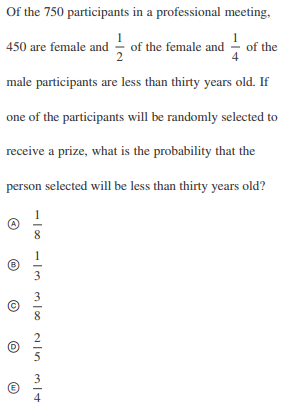Here’s a little GRE probability practice for you. In this question, we’re being asked to solve for the probability of randomly choosing a person (male or female) who is less than 30 years old.

Let’s start with what we know. There are 750 participants in total. 450 of them are explicitly described as female; therefore, we can assume 300 are male. Half of the female participants are less than 30 years old, so \$450/2=225\$. In addition, \$1/4\$ of the male participants are less than 30 years old, so \$300/4=75\$.

We now know there are 225 female participants under 30 and 75 male participants under 30. But what we want to know is the probability of choosing any person (male or female) under 30. Add these two values together to get the total number of participants under 30:

\$225+75=300\$

This tells us 300 participants are less than 30 years old. Out of a total of 750 participants, the probability of choosing a person less than 30 years old is therefore \$300/750\$.

Want to improve your GRE score by 7 points? We have the industry's leading GRE prep program. Built by world-class instructors with 99th percentile GRE scores, the program learns your strengths and weaknesses through machine learning data science, then customizes your prep program to you so you get the most effective prep possible.

But none of the answer choices above contain this particular fraction, so let’s try reducing our answer. 300 and 750 can both be divided by 150 to get \$2/5\$, which is the same as answer choice D.## 3 Tips for Approaching GRE Probability Questions

Probability doesn’t make up a particularly large part of the GRE, but you’re bound to have at least a couple of probability questions scattered throughout the test. Here are some of our best tips for approaching GRE probability questions.

### #1: Confirm the Focus is Probability

Before being able to solve a probability question, you must first be able to successfully identify one on the test. A majority of GRE probability questions use the term “probability” in their descriptions. If you’re not finding this word, though, look for other probability-related terms and phrases in the description. Common ones include:

• Event
• Outcome
• Randomly selected

It can be tempting to move swiftly on test day, but make sure you’re paying attention to what the questions are asking you to solve. One possible mistake you might make is confusing a proportion question for a probability question. Proportion questions are arithmetic-based and concentrate heavily on ratios and percentages — not probability. But these two types of questions can take similar shapes on the GRE. For example: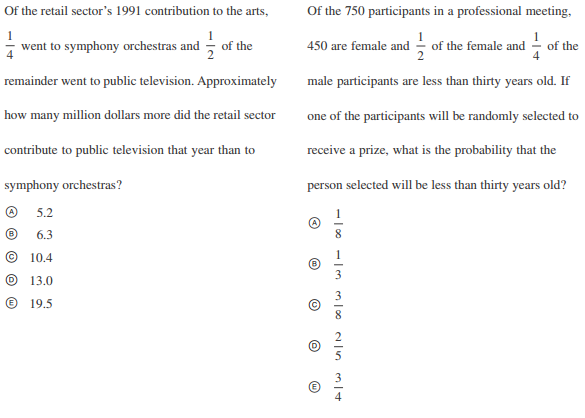At a glance, the two questions above look as though they’re probably dealing with identical concepts. After all, both are word-based questions containing fractions. Additionally, the first question offers decimals for answer choices (which could signify probability), and the second question offers fractions for answer choices (which could also signify probability).

But look more closely and you’ll see the first question is in fact focused entirely on proportions and arithmetic. There is no mention of probability or any key probability-related terms or phrases. The second question, on the other hand, uses the phrase “randomly selected” and is asking you to find a specific probability.

In the end, always make sure you are reading the questions diligently and identifying keywords so that you know exactly what you’ll be solving.

### #2: Determine a Direction

Another strategy you can use is to determine a direction. Here’s what I mean: many probability questions expect you to solve for the probability of an event. Others, however, might ask you to work backward, starting with a probability and expecting you to instead solve for a specific number, such as the total number of people in a group or a certain number of items in a sample space.

To determine which direction to take in a probability problem, look at both the description and answer choices. If you are given a total number of items in a sample space from which something has been randomly selected, you will likely solve for the probability of choosing something. If, however, you start with a probability, you’ll likely have to work backward to solve for a specific number or amount.

Take the following example: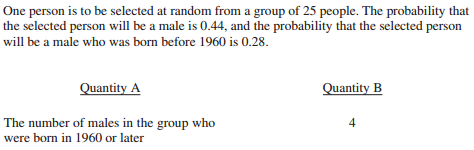In this Quantitative Comparison question, we’re being asked to compare an unknown value with the number 4. Quantity A is not a probability but rather a number of specific people in a group. So instead of solving for a probability, we’ll be working backward, using the probabilities given to us in the description to look for the number of males born in 1960 or later.

Let’s begin. The problem states there are 25 people in a group. If the probability of a male being selected is 0.44, we can use this information to figure out how many males there are in total. Remember, decimals are essentially the same as fractions. If the total is 25 people, our probability of choosing a male is therefore \$x/25\$, which should, when converted to a decimal, equal 0.44. Here’s how to set it up:

\$x/25=0.44\$

Now, solve for x:

\$x/25=0.44\$

\$x=0.44*25\$

\$x=11\$

According to our calculations, there are 11 total males in this particular group of 25 people. Now, let’s look at the males born before 1960. The probability that a male chosen was born before 1960 is 0.28 (out of a total of 25 people, not 11 males). Mimic the procedure above to calculate how many males in the group were born before 1960. Here, we’ll use a different variable, y, to represent the number of males born before 1960:

\$y/25=0.28\$

\$y=0.28*25\$

\$y=7\$

We now know 7 of the 11 males in the group were born before 1960. But the question is asking us for the number of males born in or after 1960. We can use subtraction to get the answer:

\$11-7=4\$

4 males were born in or after 1960. Because 4 is equal to Quantity B, the correct answer is C: The two quantities are equal.### #3: Plug In Numbers

For the most part, you should be able to solve GRE probability questions using a combination of algebra and arithmetic. But some questions might be purposely vague or complicated. In these situations, plugging in numbers is a good way to either solve for an answer or double-check an answer you’ve come up with using a different method.

Let’s look back at the sample question above on three mutually exclusive outcomes (p, \$p/2\$, and \$p/4\$):

Want to improve your GRE score by 7+ points?

Check out our best-in-class online GRE prep program. We guarantee your money back if you don't improve your GRE score by 7 points or more.

PrepScholar GRE is entirely online, and it customizes your prep program to your strengths and weaknesses. We also feature 2,000 practice questions, official practice tests, 150 hours of interactive lessons, and 1-on-1 scoring and feedback on your AWA essays.

Check out our 5-day free trial now:Previously, we used algebra to solve for p, as all of the events are defined using the same variable. But let’s say you weren’t sure how to solve the equation you came up with. Another way of solving this problem is by plugging in each of the answer choices. We already know the probabilities of all three possible outcomes must equal 1 when added together (per the rules of probability). In algebra, this looks like:

\$p+{p/2}+{p/4}=1\$

Now, let’s start plugging in some of our answer choices. Always make sure to start with C, or whatever the middle value is, so you’ll have a rough idea of whether you should work up or down to get a higher or lower result. Here’s what the equation looks like when we plug in C (\$3/7\$) to our equation:

\${3/7}+{(3/7)/2}+{(3/7)/4}\$

\$0.43+0.21+0.11=0.75\$

If this question were on the test, you could use your on-screen calculator to solve each fraction individually before combining all of the terms. Our result is 0.75, which is pretty close to 1 but a little lower than the answer we’re looking for. So, let’s try D, which is a slightly larger fraction, and see if we can get a higher result:

\${4/7}+{(4/7)/2}+{(4/7)/4}\$

\$0.57+0.29+0.14=1\$

Answer choice D gives us 1 and is therefore the correct answer choice. Note that this is the same answer we reached previously when using algebra to solve the equation! As you can see, it doesn’t matter what method you choose — just as long as you’re able to come up with the correct answer.

## How to Study GRE Probability

Before you take the GRE, it’s a good idea to spend some time familiarizing yourself with probability concepts and practice questions. Here are some of the top study strategies we recommend for GRE probability practice.

### #1: Review Key Concepts

Many GRE probability questions will require you to use algebra and/or arithmetic in order to get an answer. Therefore, make sure you know how to do the following:

• Set up proportions
• Use cross multiplication
• Reduce fractions
• Convert a fraction into a decimal (you can use the on-screen calculator for this)
• Set up an equation with variables

Additionally, be sure you understand all of the major probability-related terms and rules defined above so you can set up equations without hassle.### #2: Memorize Formulas

Another crucial component of GRE probability practice is memorizing key probability formulas. As previously stated, there are three major probability formulas, but the one for solving the probability of a single event occurring is by far the GRE’s favorite. Once again, this formula is:

\$\$P(E)={\number \of \successful \outcomes}/{\total \number \of \outcomes}\$\$

Make sure you diligently practice this formula and understand how to use it to solve for a specific probability. On the exam, you’re not always simply solving for a probability, though; sometimes, you’ll begin with a probability and have to work backward to find a specific value. Knowing how this formula works, then, can allow you to apply it to a variety of probability problems, even if you’re not directly solving for a probability answer.

### #3: Use High-Quality Study Materials

As with any GRE topic, always stick to the highest quality study materials so you can practice realistic probability questions and familiarize yourself with how probability will appear on the GRE. Feel free to check out our top choices for GRE prep books and our summary of the best resources for GRE math practice. As always, opt for official resources whenever possible.

Furthermore, be sure to take advantage of official practice tests. With these tests, you’ll get used to how often probability appears on the exam and learn firsthand how to tackle these questions no matter when or how they come up. ETS offers six practice tests free of charge, and we cover all of the details of these tests in our in-depth guide.

## GRE Probability: Recap

Probability doesn’t take up a lot of space on the GRE, but it’s a critical concept you should spend a decent amount of time studying, especially if your ultimate goal is to maximize your Quant score.

Before sitting for the exam, be sure to memorize the most important concepts associated with probability, including all of the major terms, rules, and formulas. Most GRE probability questions will require you to apply, in one way or another, the basic probability formula, which is:

\$\$P(E) = {\number \of \successful \outcomes} / {\total \number \of \possible \outcomes}\$\$

Our comprehensive GRE probability review has given you tons of test-day tips:

• Know how to identify a probability question
• Determine whether you need to solve for a probability or work backward and solve for a specific value
• Plug in answer choices, starting with the middle answer choice (C), if you’re unsure how to use algebra to get an answer

When studying GRE probability, try to dedicate ample time to the following:

• Reviewing key concepts, including the basics of arithmetic and algebra
• Memorizing probability formulas, particularly the one for a single event
• Practicing with high-quality prep materials, including books and official GRE practice tests

Remember, your probability of scoring highly on Quant will rise the more you study and practice specific concepts, so don’t forgo GRE probability practice. Get studying and you’ll be acing probability questions in no time!

## What’s Next?

What else is on Quant besides probability? Check out our detailed math review to learn more about the myriad of topics tested on the GRE.

Need tips for studying? Take a look at our comprehensive resource for the best math practice you can get, and read all about our top strategies for using PowerPrep.

Want specific strategies for test day? If you’d like to learn how to use your scratch paper or how to use the on-screen calculator, we’ve got you covered!